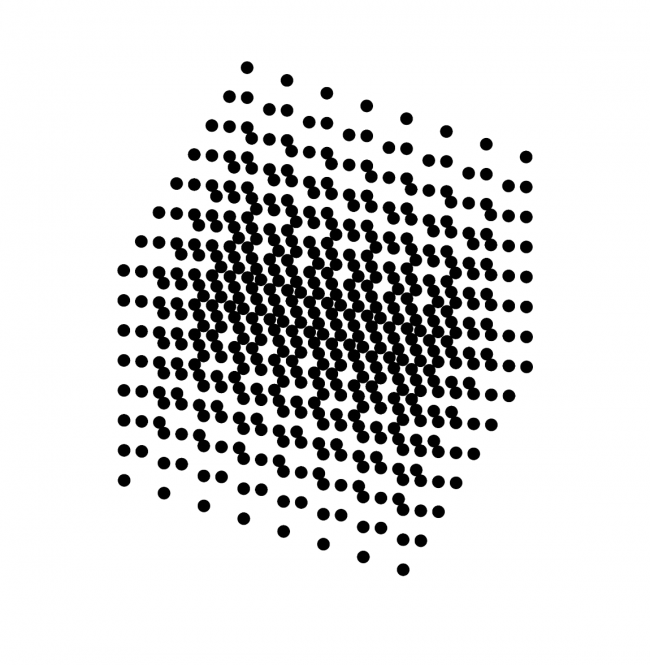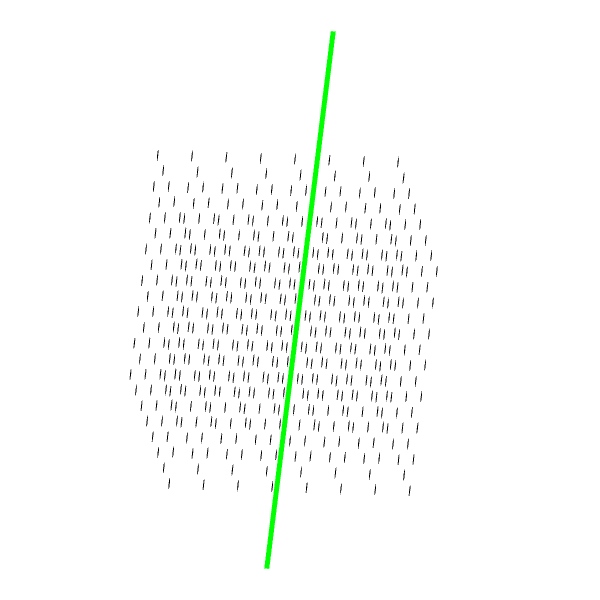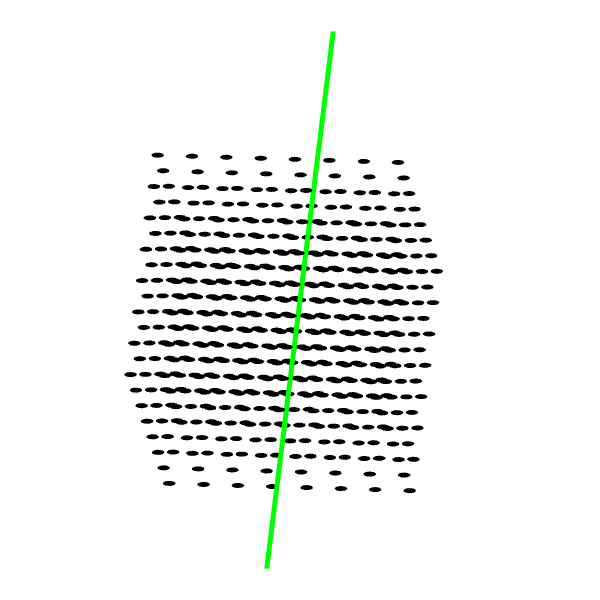#### Howdy, Stranger!

We are about to switch to a new forum software. Until then we have removed the registration on this forum.

# Replace sphere with ellipses in 3D mode

edited March 2018

The next sketch makes this:

A 3D grid with spheres, to be rotated with the use of peasy.I would, however, replace the spheres by ellipses, to make a better PDF output. But, of course, when I replace a sphere with an ellipse, the ellipse would rotate and loses the shape of a perfect circle.

What would it take to maintain the orientation of the ellipse while rotating the cube?

Thanks in advance for helping me out :-)

code:

``````import processing.pdf.*;
import peasy.*;
import peasy.org.apache.commons.math.*;
import peasy.org.apache.commons.math.geometry.*;

PeasyCam cam;

int afstand = 70;

boolean record;

void setup() {
size(600, 600, P3D);
cam = new PeasyCam(this, 10000);
noStroke();
fill(0);
ortho(-width, width, -height, height);
}

void draw() {
background(#ffffff);

if (record) {
beginRaw(PDF, "cube.pdf");
}

for (int i=0; i<8; i++) {
for (int j=0; j<8; j++) {
for (int k=0; k<8; k++) {
pushMatrix();
translate((i-3)*afstand, (j-3)*afstand, (k-3)*afstand);
sphere(10);
popMatrix();
}
}
}

if (record) {
endRaw();
record = false;
}
}

void keyPressed() {
record = true;
}
``````
Tagged:

• look at reference of PeasyCam; is there a way to receive the current angles (i guess there are 2)?

• Hmm. Just brainstorming -- I wonder if you could use screenX to retrieve 3D coordinates and then draw ellipses on a separate buffer image at the corresponding screen location.

https://processing.org/reference/screenX_.html

• Instructions:

*** Remember that in peasyCam you can double click in your sketch to reset the current cam view of your sketch. While keeping this in mid, then:

1- Use key `r` to toggle the option to show rotated ellipses or no rotation at all 2- Use key `s` to save into pdf 3- Assuming you start from init cam state, press either `x`,`y` or `z` to rotate 90 deg on that axis. You can use dbl-click to see the action of recovering your init cam orientation aka. what axis the restoration is happening on 4- Use `p` to print the current rotation matrix on the console as reported by peasycam

Kf

``````import processing.pdf.*;
import peasy.*;
import peasy.org.apache.commons.math.*;
import peasy.org.apache.commons.math.geometry.*;

PeasyCam cam;

int afstand = 70;

boolean record;
boolean rotateEllipses=false;

void setup() {
size(600, 600, P3D);
cam = new PeasyCam(this, 10000);
noStroke();
fill(0);
ortho(-width, width, -height, height);
}

void draw() {
background(#ffffff);

if (record) {
beginRaw(PDF, "cube.pdf");
}

strokeWeight(5);
stroke(0, 255, 0);
line(-width, 0, width, 0);

noStroke();
for (int i=0; i<8; i++) {
for (int j=0; j<8; j++) {
for (int k=0; k<8; k++) {
pushMatrix();
translate((i-3)*afstand, (j-3)*afstand, (k-3)*afstand);

if(rotateEllipses){
float[] rot=cam.getRotations();
rotateX(rot);
rotateY(rot);
rotateZ(rot);
}

//sphere(10);
ellipse(0, 0, 25, 10);
popMatrix();
}
}
}

if (record) {
endRaw();
record = false;
}
}

void keyPressed() {
if (key=='s')
record = true;

if (key=='p') {
println(cam.getRotations());
}

if(key=='r')
rotateEllipses=!rotateEllipses;

if (key>='x' && key<='z') {

float[] rot=cam.getRotations();
//switch(key) {
//case 'x':
//  rot=HALF_PI;
//  break;
//case 'y':
//  rot=HALF_PI;
//  break;
//case 'z':
//  rot=HALF_PI;
//  break;
//}

//MORE compact than above
rot[key-'x']=HALF_PI+0.05;
cam.setRotations(rot, rot, rot);
}
}
``````• But the OP wants the ellipses to always face the front, like billboard sprites.

Whenever I've done this I use a 2d renderer and do the 3d rotation calculations myself and just use the X and y coords of the result. You're using ortho so there's no perspective to worry about. You won't be able to use peasycam though.

• I wonder if you could use PeasyCam as the interface, translate to get the xyz coordinates at 0,0,0 -- and then retrieve a 2D x and y for each using e.g.

``````ellipse(screenX(0,0,0), screenY(0,0,0), 20, 20);
``````

The catch is:

In order to get flat/ortho results you would then need to draw the ellipses onto a 2D PGraphics -- not into the P3D space. So the base sketch is P3D with PeasyCam interface, and the output buffer is default Java2D. However I am not sure how/if rendering a PGraphics interacts with the PDF beginRaw().

• Thank you all so much for the tips. Kfrajer's post was really helpful.

@jeremydouglass I have been thinking about that kind of solution, but I wouldn't know how to plot it correctly. Also, Kfrajer's solution was very effective for what I needed to achieve.

• there's no reason the pdf writing part has to use the interactive part of the code - just put it inside a big `if (record)` block